Brakes

Block Brakes

Introduction

This type of braking system has been generally used before the 1930's for transport systems and machinery.  It is a very simple concept and requires minimum engineering.   The brake has the disadvantage that the braking surfaces are open to the environment and are easily contaminated.  These brakes are often seen on steam traction engines, steam rollers, etc.

This brake works with friction force applied to the external surface of a rotating cylinder using a block with a surface made of a suitable friction material.  The braking effect operates if the rotation is in either direction.  Depending on the design the the braking torque is higher in one direction.  Two block brake designs are considered.

 The short block when the pressure is considered to be uniform because the contact angle is relatively small. The long block when the contact angle is above say 60o and the pressure is not uniform

Nomenclature

F = Applied Force (N)
P = Brake Power kW
T = Torque (Nm)
F = Applied Force (N)
μ = Coefficient of Friction.
b = Band width
c = distance from tensioning belt to fulcrum point
n = Rotational Speed (RPM)
p max = Maximum pressure for friction surface
θ = Brake shoe contact angle
r = Radius of brake wheel

Short Block

The maximum normal force applied by the block =

F n = p max.r θ w

The torque capacity of the brake =

Tmax = Fn μ r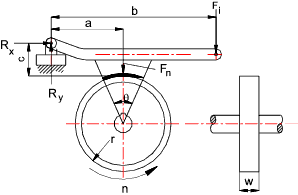Taking moments about the lever pivot point, the maximum operating force =

F i = Fn(b - μc) / a

The system as drawn is to some extent self energising because the friction force reduces the actuating force necessary.  If the pivot point is located below the brake contact point the brake would not be self sustaining

Long Block

The long block pressure distribution calculation assumes that the lever arm is rigid and the pressure is directly related to the distance from the pivot point.  The pressure is proportional to c sin θ or p = k sin θ where k is some constant.  For a specific brake material the maximum pressure = pmax.

k = p max /sin θ max... and... p = sin θ(p max /sin θ max)

The value of minimum θ is usually set to above 100 and the maximum pressure when the value of sin θ is 900. above this angle the pressure reduces.   Therefore if the maximum value of θ is less than 900 the sin θ max = sin θ. if the maximum angle is greater than 900 then sin θ max = sin 900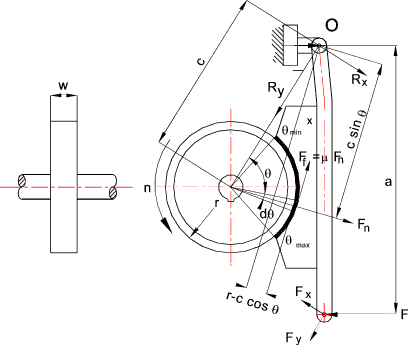To calculate the maximum braking actuating force it is necessary to take moments about the brake arm pivot point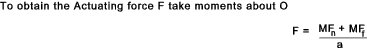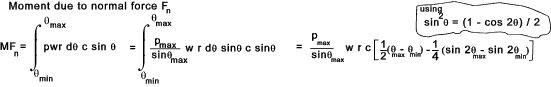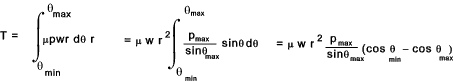The motion of the drum as shown result in an ACW friction moment and the normal reaction force of the drum also results in an ACW moment as the two moments are the same self locking cannot occur. If the rotation is reversed and the friction moment is CW then the resulting actuating force will be reduced and self-locking is possible.

To obtain the maximum torque for the brake ...Links to external brake block Design Clutches and Brakes ...A detailed selection and design guide download book Brakes .U of Western Australia - Very informative text.. Road Vehicle Brakes and Braking Notes ..Some useful notes History of Car Brakes ..Some useful information Mayr ...Brakes and Clutches

Brakes